Setup for 2 Player a Game of Food Chain Magnate

Originally generated on 1/10/2019 10:57:04 AM

Player Setup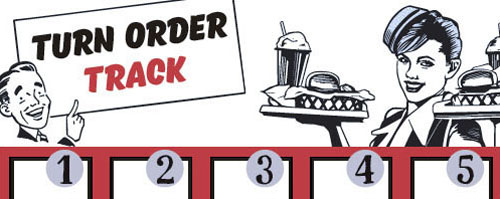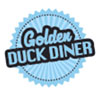Other Setup

Bank starts with \$100.00
Remove these Billboards: #12, #15, #16
Number of 1x Employee cards used = 1

Map (Balanced)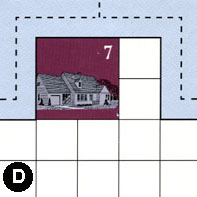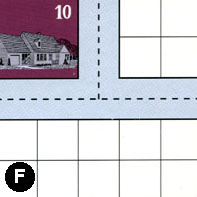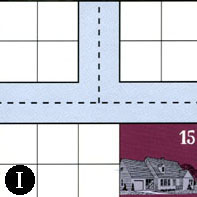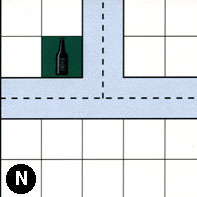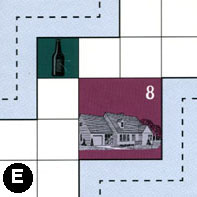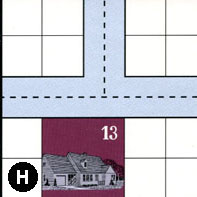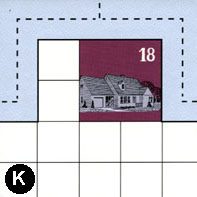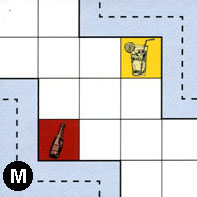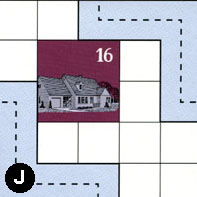Map Stats

Map Option = Balanced
Balance Issues = Not enough drink spots. Not enough large path systems. Not enough large neighborhoods. Not enough empty space in neighborhoods.
Total Number of Tiles = 9
Total Number of Starting Houses = 7
Total Number of Beer Spots = 2
Total Number of Soda Spots = 1
Total Number of Lemonade Spots = 1
Total Number of Drink Spots = 4
Number of independent path systems = 3
Number of independent neighborhoods = 12

Path System 1
Size = Medium
Number of Tiles = 9
Number of Paths = 21
Contains Loop(s) = No
Number of Starting Houses = 7
Starting Houses Pct of Map (%) = 100
Number of Beer Spots = 2
Number of Soda Spots = 0
Number of Lemonade Spots = 1
Number of Drink Spots = 3
Drink Spots Pct of Map (%) = 75
Tile Ids = D, E, F, H, I, J, K, M, N
Paths = DWE, FWN, FNE, IWN, HWN, HNE, JWS, HWE, IWE, INE, FWE, DWN, NWE, KWN, MEN, EWN, KNE, KWE, NWN, NNE, DNE What do these mean?
Starting House Ids = 0, 7, 10, 13, 15, 16, 18
Path System 2
Size = Small
Number of Tiles = 2
Number of Paths = 2
Contains Loop(s) = No
Number of Starting Houses = 2
Starting Houses Pct of Map (%) = 29
Number of Beer Spots = 0
Number of Soda Spots = 1
Number of Lemonade Spots = 0
Number of Drink Spots = 1
Drink Spots Pct of Map (%) = 25
Tile Ids = J, M
Paths = MWS, JEN What do these mean?
Starting House Ids = 0, 16
Path System 3
Size = Tiny
Number of Tiles = 1
Number of Paths = 1
Contains Loop(s) = No
Number of Starting Houses = 2
Starting Houses Pct of Map (%) = 29
Number of Beer Spots = 0
Number of Soda Spots = 0
Number of Lemonade Spots = 0
Number of Drink Spots = 0
Drink Spots Pct of Map (%) = 0
Tile Ids = E
Paths = EES What do these mean?
Starting House Ids = 8, 13

Neighborhood 1
Total Size = Small
Number of Total Spaces = 4
Number of Empty Spaces (for new houses & gardens) = 4
Number of Beer Spots = 0
Number of Soda Spots = 0
Number of Lemonade Spots = 0
Number of Drink Spots = 0
Number of Starting Houses = 0
Starting Houses Pct of Map (%) = 0
Tile Ids = B
Neighborhood 2
Total Size = Medium
Number of Total Spaces = 8
Number of Empty Spaces (for new houses & gardens) = 8
Number of Beer Spots = 0
Number of Soda Spots = 0
Number of Lemonade Spots = 0
Number of Drink Spots = 0
Number of Starting Houses = 0
Starting Houses Pct of Map (%) = 0
Tile Ids = A, B
Neighborhood 3
Total Size = Large
Number of Total Spaces = 26
Number of Empty Spaces (for new houses & gardens) = 21
Number of Beer Spots = 0
Number of Soda Spots = 1
Number of Lemonade Spots = 0
Number of Drink Spots = 1
Number of Starting Houses = 1
Starting Houses Pct of Map (%) = 14
Tile Ids = A, G, I, S
Starting House Ids = 15
Neighborhood 4
Total Size = Small
Number of Total Spaces = 4
Number of Empty Spaces (for new houses & gardens) = 4
Number of Beer Spots = 0
Number of Soda Spots = 0
Number of Lemonade Spots = 0
Number of Drink Spots = 0
Number of Starting Houses = 0
Starting Houses Pct of Map (%) = 0
Tile Ids = I
Neighborhood 5
Total Size = Medium
Number of Total Spaces = 8
Number of Empty Spaces (for new houses & gardens) = 7
Number of Beer Spots = 0
Number of Soda Spots = 0
Number of Lemonade Spots = 1
Number of Drink Spots = 1
Number of Starting Houses = 0
Starting Houses Pct of Map (%) = 0
Tile Ids = B, P
Neighborhood 6
Total Size = Medium
Number of Total Spaces = 16
Number of Empty Spaces (for new houses & gardens) = 8
Number of Beer Spots = 0
Number of Soda Spots = 0
Number of Lemonade Spots = 0
Number of Drink Spots = 0
Number of Starting Houses = 2
Starting Houses Pct of Map (%) = 29
Tile Ids = A, B, P, S
Starting House Ids = 2, 4
Neighborhood 7
Total Size = Medium
Number of Total Spaces = 8
Number of Empty Spaces (for new houses & gardens) = 8
Number of Beer Spots = 0
Number of Soda Spots = 0
Number of Lemonade Spots = 0
Number of Drink Spots = 0
Number of Starting Houses = 0
Starting Houses Pct of Map (%) = 0
Tile Ids = G, I
Neighborhood 8
Total Size = Small
Number of Total Spaces = 4
Number of Empty Spaces (for new houses & gardens) = 4
Number of Beer Spots = 0
Number of Soda Spots = 0
Number of Lemonade Spots = 0
Number of Drink Spots = 0
Number of Starting Houses = 0
Starting Houses Pct of Map (%) = 0
Tile Ids = P
Neighborhood 9
Total Size = Large
Number of Total Spaces = 32
Number of Empty Spaces (for new houses & gardens) = 25
Number of Beer Spots = 2
Number of Soda Spots = 1
Number of Lemonade Spots = 0
Number of Drink Spots = 3
Number of Starting Houses = 1
Starting Houses Pct of Map (%) = 14
Tile Ids = K, P, R, S
Starting House Ids = 18
Neighborhood 10
Total Size = Large
Number of Total Spaces = 27
Number of Empty Spaces (for new houses & gardens) = 23
Number of Beer Spots = 0
Number of Soda Spots = 0
Number of Lemonade Spots = 0
Number of Drink Spots = 0
Number of Starting Houses = 1
Starting Houses Pct of Map (%) = 14
Tile Ids = G, J, R, S
Starting House Ids = 16
Neighborhood 11
Total Size = Small
Number of Total Spaces = 4
Number of Empty Spaces (for new houses & gardens) = 0
Number of Beer Spots = 0
Number of Soda Spots = 0
Number of Lemonade Spots = 0
Number of Drink Spots = 0
Number of Starting Houses = 1
Starting Houses Pct of Map (%) = 14
Tile Ids = G
Starting House Ids = 12
Neighborhood 12
Total Size = Small
Number of Total Spaces = 4
Number of Empty Spaces (for new houses & gardens) = 4
Number of Beer Spots = 0
Number of Soda Spots = 0
Number of Lemonade Spots = 0
Number of Drink Spots = 0
Number of Starting Houses = 0
Starting Houses Pct of Map (%) = 0
Tile Ids = R

v2.1.5